Open in App
Not now

# Class 9 RD Sharma Solutions – Chapter 1 Number System – Exercise 1.2

• Last Updated : 03 Mar, 2021

### iii) 15/4

Solutions:

(i) 42/100

Using long division method: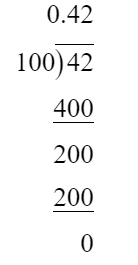So, 42/100 = 0.42

(ii) 327/500

Using long division method: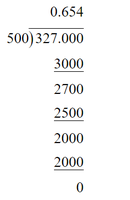So, 327/500 = 0.654

(iii) 15/4

Using long division method: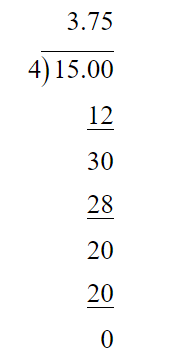So, 15/4 = 3.75

### (vi) 33/26

Solutions:

(i) Using long division method: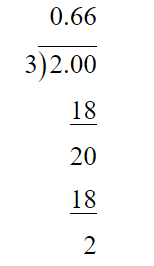So, 2/3 = 0.66.. =(ii) Using long division method: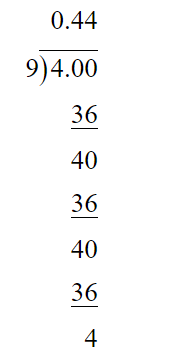So, -4/9 = -0.44.. =(iii) Using long division method: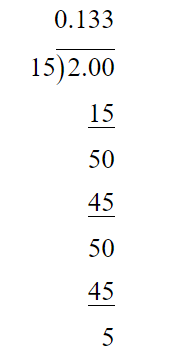So, -2/15 = (- 0.133..) =(iv) -22/13 = -(1 + 9/13)

Using long division method: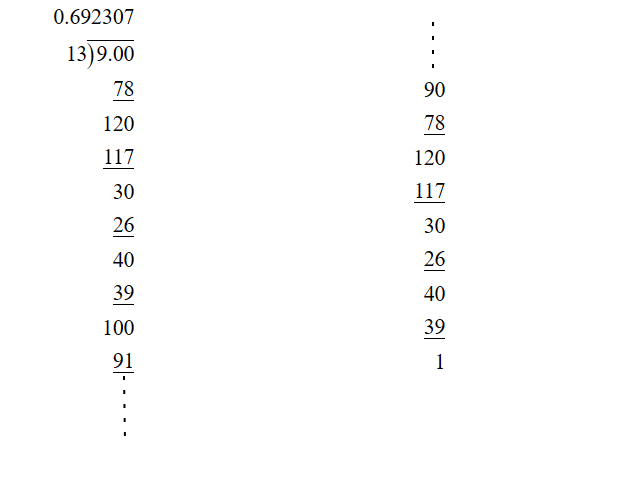So, -22/3 = -(1.692307…) =(v) 437/999

Using long division method: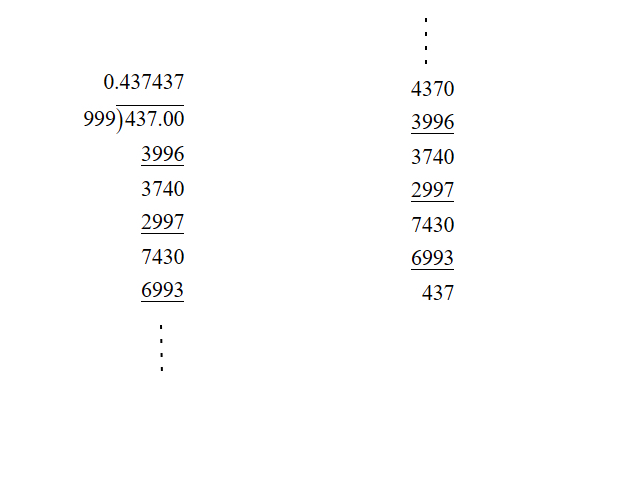So, 437/999 = 0.437… =(vi) 33/26 =Using long division method: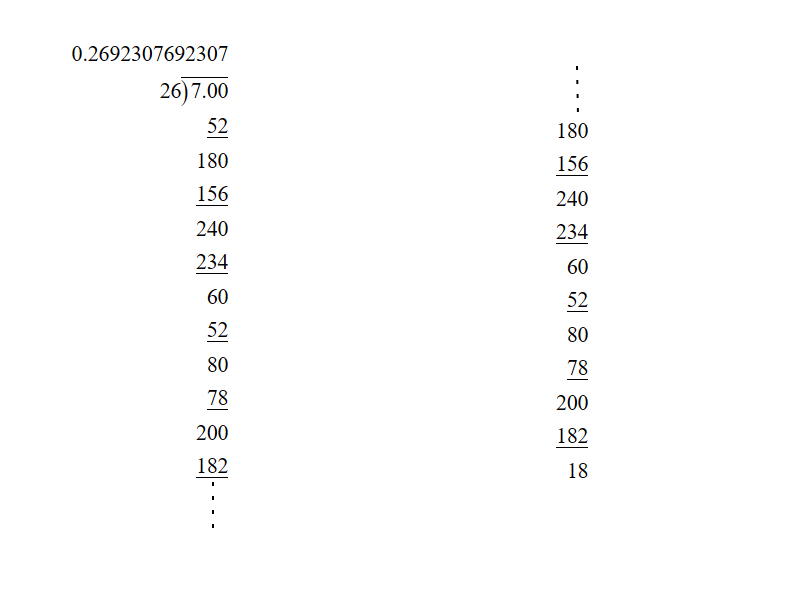So, 33/26 = 1.2692307… =### Question 3. Look at several examples of rational numbers in the form p/q (q ≠ 0), where p and q are integers with no common factors other than 1 and having terminating decimal representations. Can you guess what property q must satisfy?

Solution:

p/q will be terminating decimal when prime factorization of q must have factors 2 or 5 or both.

My Personal Notes arrow_drop_up
Related Articles